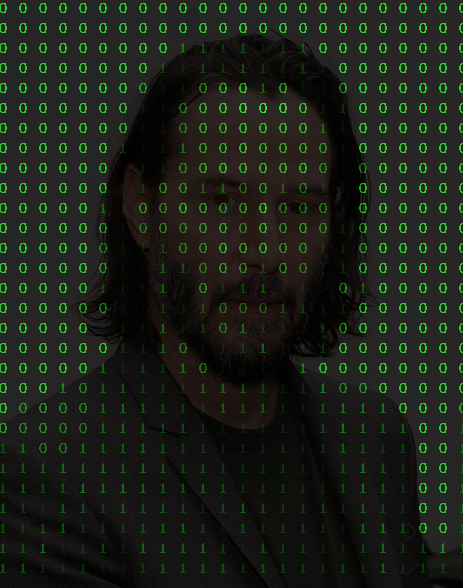### 准备工作`12345` `````` org.bytedeco opencv-platform 4.5.3-1.5.6 ``````

### 开始写代码

 `12` `````` val path = "path/to/jinu.jpg" val img = opencv_imgcodecs.imread(path) ``````

 `123456` `````` val size = img.size() val height = size.height() val width = size.width() val cellHeight = 20 val cellWidth = 20 ``````

 `123456789` `````` val img2 = img.clone() opencv_imgproc.resize( img, img2, Size(width / cellWidth, height / cellHeight), 0.0, 0.0, opencv_imgproc.INTER_NEAREST ) ``````

 `1` ``````val newImg = Mat.zeros(height, width, opencv_core.CV_8UC3).asMat() ``````

 `1234567891011121314151617` `````` val indexer = img2.createIndexer() val bgr = IntArray(3) for (i in 0 until indexer.rows()) { for (j in 0 until indexer.cols()) { indexer.get(i, j, bgr) val b = bgr val g = bgr val r = bgr val k = (b + g + r) / 3 if (k < 128) { opencv_imgproc.putText(newImg,"1", Point(j.toInt()*cellWidth, i.toInt()* cellHeight), opencv_imgproc.FONT_HERSHEY_COMPLEX_SMALL, 0.7, Scalar(0.0,g.toDouble(),0.0, 0.0)) } else { opencv_imgproc.putText(newImg,"0", Point(j.toInt()*cellWidth, i.toInt()* cellHeight), opencv_imgproc.FONT_HERSHEY_COMPLEX_SMALL, 0.7, Scalar(0.0,g.toDouble(),0.0, 0.0)) } } } ``````

 `1` ``````addWeighted(img, 0.2, newImg, 0.8, 0.0, img) ``````### 完整代码

 `123456789101112131415161718192021222324252627282930313233343536373839404142434445464748495051525354555657585960` ``````import org.bytedeco.javacpp.indexer.UByteIndexer import org.bytedeco.opencv.global.opencv_core import org.bytedeco.opencv.global.opencv_core.addWeighted import org.bytedeco.opencv.global.opencv_highgui import org.bytedeco.opencv.global.opencv_imgcodecs import org.bytedeco.opencv.global.opencv_imgproc import org.bytedeco.opencv.opencv_core.Mat import org.bytedeco.opencv.opencv_core.Point import org.bytedeco.opencv.opencv_core.Scalar import org.bytedeco.opencv.opencv_core.Size fun main() { val path = "/Users/wbf/Desktop/jinu.jpg" val img = opencv_imgcodecs.imread(path) val size = img.size() val height = size.height() val width = size.width() val cellHeight = 20 val cellWidth = 20 val img2 = img.clone() opencv_imgproc.resize( img, img2, Size(width / cellWidth, height / cellHeight), 0.0, 0.0, opencv_imgproc.INTER_NEAREST ) val newWidth = img2.size().width() val newHeight = img2.size().height() val newImg = Mat.zeros(height, width, opencv_core.CV_8UC3).asMat() val indexer = img2.createIndexer() val bgr = IntArray(3) for (i in 0 until indexer.rows()) { for (j in 0 until indexer.cols()) { indexer.get(i, j, bgr) val b = bgr val g = bgr val r = bgr val k = (b + g + r) / 3 if (k < 128) { opencv_imgproc.putText(newImg,"1", Point(j.toInt()*cellWidth, i.toInt()* cellHeight), opencv_imgproc.FONT_HERSHEY_COMPLEX_SMALL, 0.7, Scalar(0.0,g.toDouble(),0.0, 0.0)) } else { opencv_imgproc.putText(newImg,"0", Point(j.toInt()*cellWidth, i.toInt()* cellHeight), opencv_imgproc.FONT_HERSHEY_COMPLEX_SMALL, 0.7, Scalar(0.0,g.toDouble(),0.0, 0.0)) } } } addWeighted(img, 0.2, newImg, 0.8, 0.0, img) opencv_highgui.imshow("image", img) opencv_highgui.waitKey(0) } ``````

### 总结